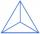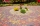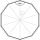# Hexagon cut pyramid

Calculate the volume of a regular 6-sided cut pyramid if the bottom edge is 30 cm, the top edge us 12 cm, and the side edge length is 41 cm.

Result

V =  4593.916 cm3

#### Solution:Leave us a comment of example and its solution (i.e. if it is still somewhat unclear...):Be the first to comment!#### To solve this example are needed these knowledge from mathematics:

Pythagorean theorem is the base for the right triangle calculator. See also our trigonometric triangle calculator.

## Next similar examples:

1. Tetrahedral pyramidIt is given a regular tetrahedral pyramid with base edge 6 cm and the height of the pyramid 10 cm. Calculate the length of its side edges.
2. 4s pyramidRegular tetrahedral pyramid has a base edge a=17 and collaterally edge length b=32. What is its height?
3. TetrahedronWhat is the angle of the sides from the base of a three-sided pyramid where the sides are identical?
4. MedianIn triangle ABC is given side a=10 cm and median ta= 13 cm and angle gamma 90°. Calculate length of the median tb.
5. PavementCalculate the length of the pavement that runs through a circular square with a diameter of 40 m if distance the pavement from the center is 15 m.
6. Regular n-gonIn a regular n-angle polygon the internal angle is 144 degrees. Find the number n indicating the number of sides of this polygon.
7. Reference angleFind the reference angle of each angle:
8. Center traverseIt is true that the middle traverse bisects the triangle?
9. A truckA truck departs from a distribution center. From there, it goes 20km west, 30km north and 10km west and reaches a shop. How can the truck reach back to the distribution center from the shop (what is the shortest path)?
10. Holidays - on poolChildren's tickets to the swimming pool stands x € for an adult is € 2 more expensive. There was m children in the swimming pool and adults three times less. How many euros make treasurer for pool entry?The double ladder shoulders should be 3 meters long. What height will the upper top of the ladder reach if the lower ends are 1.8 meters apart?The double ladder is 8.5m long. It is built so that its lower ends are 3.5 meters apart. How high does the upper end of the ladder reach?We want to prove the sentence: If the natural number n is divisible by six, then n is divisible by three. From what assumption we started?In a rectangular trapezoid ABCD with right angles at vertices A and D with sides a = 12cm, b = 13cm, c = 7cm. Find the angles beta and gamma and height v.I have a wall 2m high. I need a 15 degree angle (upward) to second wall 4 meters away. How high must the second wall?Circular reflector throws light cone with a vertex angle 49° and is on 33 m height tower. The axis of the light beam has with the axis of the tower angle 30°. What is the maximum length of the illuminated horizontal plane?The ladder is 10 m long The ladder is 8 m high How many meters is the distant heel from the wall?